All submissions of the EM system will be redirected to Online Manuscript Submission System. Authors are requested to submit articles directly to Online Manuscript Submission System of respective journal.

# Original Article

, Volume: 5( 3)

## Gravitational Properties of Atom

*Correspondence:
S Orlov Petrozavodsk State University, Russia, Tel: 0542 236 8558; E-mail: [email protected]

Received Date: June 29, 2017 Accepted Date: August 24, 2017 Published Date: September 06, 2017

Citation: Orlov S. Gravitational Properties of Atom. J Phys Astron. 2017;5(3):118

### Abstract

The evidence that the strength of any body provide nuclear forces of gravity. The nuclear force of gravity generated by the essential micro vortices. The vortex creates a pressure gradient in the ether. The pressure gradient is the source of nuclear energy. The nuclear force of gravity on the surface of the cores is equal for all elementary particles.

### Keywords

Theory of atomic vortex gravitation; Cosmology and cosmogony; Strength; Mass and energy substances

### Introduction

The strength of any substance is created interatomic forces of attraction. In modern science these are called electrostatic or quantum-mechanical. The nature of the interatomic forces, there are following types of communication in solids: ionic, covalent, metallic, hydrogen bond, Van der Waals forces .

In this paper we propose to consider a new model of the interatomic bonds. The interatomic forces of attraction is the atomic force of gravity. The proposed physical model is based on the theory of vortex gravitation, cosmology and cosmogony. The next chapter presents the basic principles of the theory of vortex gravitation.

### Vortex Gravity

In the theory, the vortex gravitation, cosmology and cosmogony states that outer space is of ether vortices. These vortices (torsion bars) created: Celestial bodies (objects) and elementary particles, The force of attraction between them. Vortices and the corresponding body of different value. The largest terrestrial vortex, which can observe people it's a universal vortex, the smallest nuclear. The orbital speed of ether in each vortex decreases toward the periphery of the vortex according to the inverse square law. Speed of the ether is several orders of magnitude greater than the speed of light. The change of orbital velocity in a closed volume ether create in this volume is inversely proportional to the change in pressure. Reducing the pressure in a whirlwind, forcing all of the bodies, according to the law of Archimedes, to move in the direction of least pressure, that is, to the center of the torsion bar (atom). These Archimedian forces are the forces of gravity. Such a pattern acts the same in all essential vortices. The ether is super small dense gas. It consists of the smallest particles – amers [2,3]. Ether freely permeates any body or substance, except for super-dense bodies, which are the nuclei of atoms. Therefore, according to the law of Archimedes, the vortex gravitation force do not act on the body mass. These forces act on the total amount of nucleons in the body, which is in the vortex flow ether.

According to the vortex cosmogony, the atoms was created micro vortices 1st order. In the center of atom vortex gravitational force achieves huge quantities. This force of gravity produces the same huge compressive force on ether's substances (amers) in the center of the vortex. Therefore, the substance in the center of the atomic vortex is condensed to the limit values and ether cannot penetrate it. This center is the nucleus of the atom. By the rotation of the ether near the surface of the nucleus, it acts the resistance of this surface. Therefore, on the lower orbits of the atomic vortex, turbulence occurs and vortices even smaller 2nd order. Micro vortices 2nd order less micro vortices 1st order to an enormous value. Micro vortices 2nd order creates ultrafine particles of appropriate size. These particles create the substance of the atom, which surrounds the nucleus of the atom.

As the distance from the atomic nucleus vortex gravitation force decreases.

Therefore, the substance of the atom, outside of its nucleus, also reduces its density is proportional to the force of gravity and the distance from the center of the atom. Consequently, the flow of atomic vortex freely permeates the matter outside the nucleus of an atom. Based on the above it can be concluded that the force of atomic attraction begins to have effect from the surface of the atomic nucleus and decreases in accordance with the classical law of inverse square distance from the center of the atom.

In modern physics it is argued that the density of substance nucleons is the same in all elementary particles [4,5]. This claim corresponds to the proposed atomic model. As mentioned above, the density of the nucleus and the force of compression or force of gravity on the surface of the nuclei should be maximum and equal for all atoms (substances).

In the theory of vortex gravitation  derived the following equation to determine the strength of the vortex gravitation, including atomic: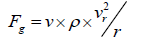(1), from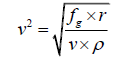(2), where

V – volume of nucleons, which is the strength of the vortex gravitation,

Vr – the speed of the orbital circulation of ether on a given orbit,

r – the radius of the orbit, which is determined by the strength of the vortex gravitation,

ρ =8.85 × 10-12 kg/ m3 is the density of ether .

The above proposed gravitational principle of creation and the existence of atoms and atomic nucleus in modern science is not studied. Consequently, there is no calculation principles of interatomic forces of attraction based on the concept of gravity.

The following chapter provides a review of the calculation of atomic forces of gravity. Interatomic attraction force is determined, not energy .

### The Nuclear Gravity

The strength of the vortex, atomic gravity depends on the speed of rotation of the whirlwind atomic ether (Equation 1). We define this rate for an atomic, etheric vortex uranium, based on experimental properties.

1. The limit tensile strength of uranium σB=37 ??/??

2. The number density of uranium ρu=19100 ??/?3

3. The mass of an atom of uranium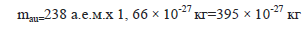4. The number of atoms in 1 m3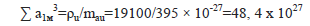5. The number of atoms in the plane of the cross section of uranium with an area of 1 m26. The same in-plane area of 1 mm2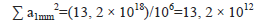7. The limit of tensile strength for a single atom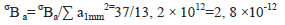8. Assuming that the gravitational force of atoms Faa equivalent tensile strength of the substance, taking into account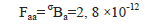9. The number of atoms in the linear dimension of 110. The interatomic distance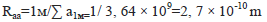11. The mass number of the nucleus A=395, 1

12. The radius of the nucleus of an atom13. The volume of the nucleus of an atom14. Substitute in equation (2) known values VA, Raa, ρ. Determine the speed of the ether vr on the interatomic distance (orbit Raa).On the basis of the relationship between the orbital velocity of the ether from the radius of the orbit of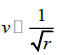determined by the speed of rotation of the ether on the surface of the nucleus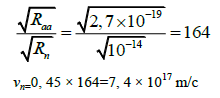15. Orbital velocity of the ethercreates the following the strength of the vortex gravitation on the interatomic distance16. Defined vortex force of gravity on the surface of the nucleus, based on the inverse square law of distance Fn/Fa=Ra/Rn :The same scheme is defined the force of gravity on the surface of the atomic nuclei for other substances. The results are shown in Table 1

Name Gold Coppe Silver Uranium Platinum
Fn 2, 1 × 1038  × VA 1, 2 × 1039 × VA 4, 5 × 1038 × VA 4, 7 × 1038 × VA 3.4 ×1038  × VA

Table 1. The atomic value of gravity at the surface of nuclei of various substances (TABLE 1) equal, in the same order.

Note 1

The calculation of gravitational forces of an atom of uranium-not complete and not accurate as it does not include the gravitational force from other neighboring atoms and are based on experimental values.

Note 2

The calculation of gravity, atomic forces on the classical laws of gravity (Newton's theory and Einstein's) is impossible, since in these theories the source of gravitational forces are the masses of the bodies, measured in kilograms. Therefore, the results of calculations according to the classical equations differ from the actual values tremendously. For example determine the force of attraction between the atoms of uranium on the basis of the equation of universal gravitation Newton:(3)

On the basis of Newton's equations the magnitude of the nuclear gravitation (1, 4 × 10-40) to 1028 times smaller than the actual, experimental (2.8 × 10-12). Thus known classical gravitation theory for the study of microparticles cannot be used. So have any new concepts interatomic attraction.

### Nuclear Energy

The velocity gradient of ether in the nuclear whirlwind (the torsion bar) creates not only the force of gravity, but acts as a source of energy. The article "the equivalence of energy and mass of atomic energy" on the basis of the equation Mendeleev-Clayperon shows a property of a gaseous medium (ether) with the pressure to create energy. Selected energy E is determined by the equation:(4), from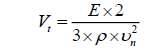(5), where

vn=7 × 1017 – maximum speed of air in the atomic vortex ( on the surface of the nucleus).

Vt – volume of ethereal atomic vortex

ρ =8.85 × 10-12 kg/ m3 is the density of the ether

We define the energy value of the uranium atom using an experimental Poincare formula.

E=m c2=3, 95 × 10-25 × 9 × 1017=3, 55 × 10-7 (J ) (6)

m=3, 95 × 10-25 kg is the mass of the uranium atom

c=3 × 108 m/c is the speed of light

Substitute the numerical values of E, ρ, Vn in equation (5), we define the atomic volume, essential torsion.(7)

In the theory of vortex gravitation the proof that gravity, ethereal vortices have a disc-like configuration. Therefore, the obtained volume of the torsion in equation (7) corresponds to its size with the size of an atom. In particular, it can be essential torsion bar with a radius of 10-8 m and the axial thickness of 10-10 m. Therefore, the equation for determining the energy on the basis of the vortex gravitation (3) corresponds to the experimental formula (6).

Note 3

With the destruction of (splitting) of the atom (nucleus) is destroyed and atomic torsion. The pressure in torsion increases sharply to the values of free ether, which must be accompanied by the release of energy, according to the formula (6).

### Conclusion

Based on the above conclusions can be drawn: The strength of all bodies providing nuclear forces of gravity rather than electrostatic or quantum-mechanical. The interatomic forces of attraction can only be determined with the use of equations and the theory of vortex gravitation. The force of atomic attraction on the surface of the cores is the same for all atoms. The gravitational mass of a body is equivalent to the volume of the nucleons in the body. The proposed calculations for the first time obtained a result that proves that the interatomic attraction, the mass of the nucleus (the amount of nucleons) and the energy of these atoms generates the same physical process, the rotation of the ether, the atomic vortex.

### References

Welcome Message

Select your language of interest to view the total content in your interested language

###### Citations : 260

Journal of Physics & Astronomy received 260 citations as per Google Scholar report

#### Indexed In

• Open J Gate
• China National Knowledge Infrastructure (CNKI)
• Cosmos IF
• Directory of Research Journal Indexing (DRJI)
• MIAR
• Secret Search Engine Labs
• Euro Pub
• clarivate-web-of-science-logo-vector

### 9th International Conference on Physics

Edinburgh, Scotland

### 6th International Conference on Astronomy, Astrophysics and Space Science

Budapest, Hungary

Berlin, Germany

### 9th International Conference on Physics

Edinburgh, Scotland

### 6th International Conference on Astronomy, Astrophysics and Space Science

Budapest, Hungary

### World Congress on Quantum Physics

Berlin, Germany

#### For Librarians#### Open Access Journals